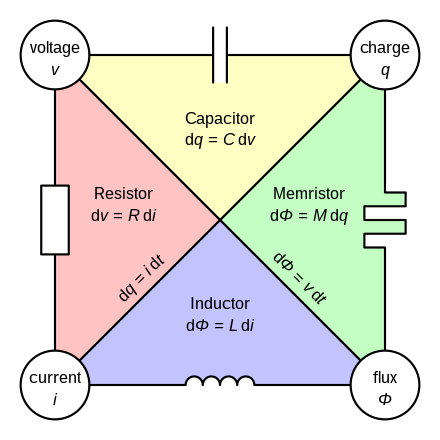400-088-6921155-8888-6921#### 产品分类# 忆阻器

（memristor）又名记忆电阻（memory resistor的混成词），是一种被动电子元件。如同电阻器，忆阻器能产生并维持一股安全的电流通过某个装置。但是与电阻器不同的地方在于，忆阻器可以在关掉电源后，仍能“记忆”先前通过的电荷量。两组的忆阻器更能产生与晶体管相同的功能，但更为细小。最初于1971年，加州大学伯克利分校的蔡少棠教授根据电子学理论，预测到在电阻器、电容器及电感元件之外，还存在电路的第四种基本元件，即是忆阻器。目前正在开发忆阻器的团队包括惠普、SK海力士、HRL实验室。

之后从2000年始，研究人员在多种二元金属氧化物和钙钛矿结构的薄膜中发现了电场作用下的电阻变化，并应用到了下一代非挥发性内存-阻抗存储器（RRAM或ReRAM）中。2008年4月，惠普公司公布了基于TiO2的RRAM器件，并首先将RRAM和忆阻器联系起来。但目前仍然有专家认为，这些实作出的电路，并不是真正的忆阻器。

## 背景在蔡少棠教授1971年的论文中，他外推了对称的非线性电阻（电压与电流），非线性电容器（电压与电荷），和非线性电感（磁通量与电流）之间的的概念，并推断出忆阻器作为一个类似于基本的非线性电路元件的，连接磁链和电荷。对比线性（或非线性）的电阻，忆阻器有一个动态的包括过去的电压或电流的记忆的电流和电压之间的关系。其他科学家已经提出动态记忆电阻器，例如伯纳德建模的存储电阻器，但是，蔡试图引进数学一般性。

## 理论在忆阻器中，磁通量（{\displaystyle\Phi _{\mathrm{B}}}\Phi_\mathrm B）受到累积的电荷（q）所影响。磁通量按电荷的改变率称之为“忆阻值”：

{\displaystyle M(q)={\frac{\mathrm{d}\Phi _{\mathrm{B}}}{\mathrm{d}q}}}M(q)=\frac{\mathrm d\Phi_\mathrm B}{\mathrm dq}

故此忆阻值可以与其余三种基本的电子元件作出比较：

电阻：{\displaystyle R(I)={\frac{\mathrm{d}V}{\mathrm{d}I}}}R(I)=\frac{\mathrm dV}{\mathrm dI}

电感：{\displaystyle L(I)={\frac{\mathrm{d}\Phi _{\mathrm{B}}}{\mathrm{d}I}}}L(I)=\frac{\mathrm d\Phi_\mathrm B}{\mathrm dI}

电容：{\displaystyle{\frac{1}{C(q)}}={\frac{\mathrm{d}V}{\mathrm{d}q}}}\frac{1}{C(q)}=\frac{\mathrm dV}{\mathrm dq}

当中{\displaystyle q}q是电荷；{\displaystyle I}I是电流；{\displaystyle V}V是电压；而{\displaystyle\Phi _{\mathrm{B}}}\Phi_\mathrm B则是磁通量。

根据法拉第电磁感应定律及复合函数求导法则，可见忆阻器的电压V是与电流I及忆阻值的积有关：

{\displaystyle V(t)=M(q(t))I(t)\,}V(t)=M(q(t))I(t)\,

由此可见，忆阻器可以成为一个电阻器。但是“电阻”的M(q)会随累积的电荷而改变。忆阻值可以说是随流经忆阻器的电流历史所改变，彷如在电容器的电压一般。

忆阻器的行为是类似的其他三个基本组成部分。

电荷({\displaystyle q}q)电流({\displaystyle I}I)

电压({\displaystyle U}U)电容(倒数)

{\displaystyle{\frac{1}{C}}={\frac{\mathrm{d}U}{\mathrm{d}q}}={\frac{\mathrm{d}{\dot{\Phi}}}{\mathrm{d}q}}}\frac{1}{C}=\frac{\mathrm{d}U}{\mathrm{d}q}=\frac{\mathrm{d}\dot\Phi}{\mathrm{d}q}

电阻率

{\displaystyle R={\frac{\mathrm{d}U}{\mathrm{d}I}}={\frac{\mathrm{d}{\dot{\Phi}}}{\mathrm{d}{\dot{q}}}}}R=\frac{\mathrm{d}U}{\mathrm{d}I}=\frac{\mathrm{d}\dot\Phi}{\mathrm{d}\dot{q}}

磁通量({\displaystyle\Phi}\Phi)忆阻器

{\displaystyle M={\frac{\mathrm{d}\Phi}{\mathrm{d}q}}}M=\frac{\mathrm{d}\Phi}{\mathrm{d}q}

电感

{\displaystyle L={\frac{\mathrm{d}\Phi}{\mathrm{d}I}}={\frac{\mathrm{d}\Phi}{\mathrm{d}{\dot{q}}}}}L=\frac{\mathrm{d}\Phi}{\mathrm{d}I}=\frac{\mathrm{d}\Phi}{\mathrm{d}\dot{q}}

## 实现

Intel与Micron联合研发的3D XPoint。（Intel表示所用并不是ReRAM，根据推断，为相变存储器的可能性更大，此条消息存疑。）厂商表示，此技术的密度是DRAM的十倍、速度是NAND的千倍、写入次数为10,000,000次。

## 潜在应用

威廉姆斯的固态的忆阻器可以组合成所谓交叉开关锁存器的设备，这可能会取代晶体管建造未来的电脑，占用面积小得多。

## 种类

忆阻器具有电化电池表现的特征。

2007年惠普公司资讯与量子系统实验室的研究人员在理查德·斯坦利·威廉姆斯的领导下成功研制了固态的忆阻器－它是由一片双层的二氧化钛薄膜所形成，当电流通过时，其电阻值就会改变。固态的忆阻器的制造需要涉及物料的纳米技术。这个忆阻器并不像其理论般涉及磁通量，或如电容器般储存电荷，而是以化学技术来达至电阻随电流历史改变的性质。

不过，三星集团却有一项正申请专利的忆阻器，采用了类似惠普公司的技术。故此谁是忆阻器的创始人则有待澄清。

目前(2008)惠普公司是以两层二氧化钛薄膜来制作忆阻器元件，其中一层掺杂。

## 可能应用

其元件特性，适合模拟神经元突触的部分运作，使得电脑神经网络制作上更能接近人脑。

威廉姆斯的固态忆阻器可以组合成晶体管，尽管小得多。They can also be fashioned into non-volatile solid-state memory,which would allow greater data density than hard drives with access times potentially similar to DRAM,replacing both components.HP has reported that its version of the memristor is about 10 times slower than DRAM

Some patents related to memristors appear to include applications in programmable logic,signal processing,neural networks,and control systems.

### 相关新闻

##### 联系方式

15588886921

400热线

400-0886921

2466458158@qq.com

##### 二维码